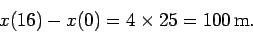Next: Worked example 2.2: Speed Up: Motion in 1 dimension Previous: Free-fall under gravity

## Worked example 2.1: Velocity-time graph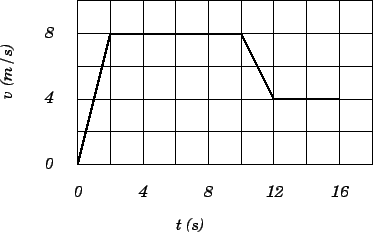Question: Consider the motion of the object whose velocity-time graph is given in the diagram.
1. What is the acceleration of the object between times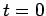and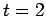?
2. What is the acceleration of the object between times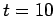and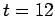?
3. What is the net displacement of the object between timesand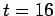?

1. The-graph is a straight-line betweenand, indicating constant acceleration during this time period. Hence,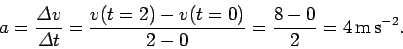2. The-graph is a straight-line betweenand, indicating constant acceleration during this time period. Hence,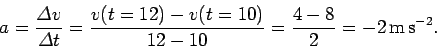3. Now,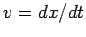, so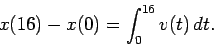In other words, the net displacement between timesandequals the area under the-curve, evaluated between these two times. Recalling that the area of a triangle is half its width times its height, the number of grid-squares under the-curve is 25. The area of each grid-square is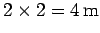. Hence,